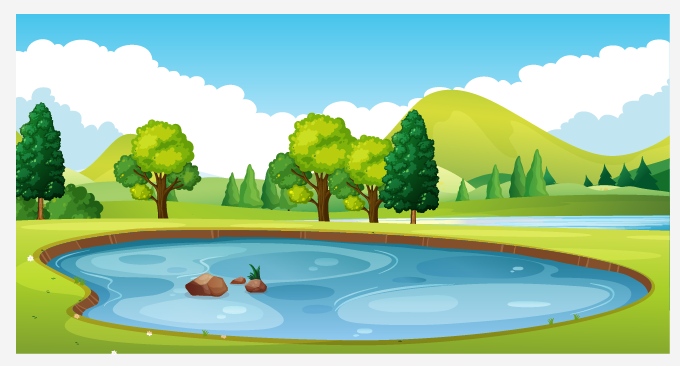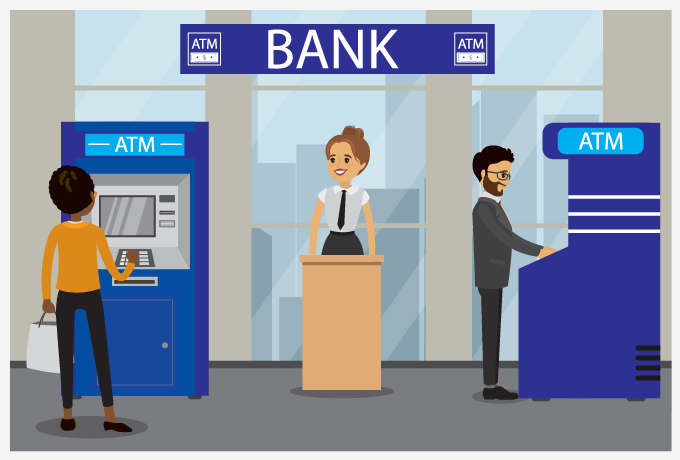# Rounding To The Nearest Hundredth - Definition with Examples

The Complete K-5 Math Learning Program Built for Your Child

• 40 Million Kids

Loved by kids and parent worldwide

• 50,000 Schools

Trusted by teachers across schools

• Comprehensive Curriculum

Aligned to Common Core

## Rounding to the Nearest Hundredth

Rounding is a process in which we convert a given number into an easy number for various purposes. This easy number is not the exact value but is an approximate value of our number.

## Purpose of rounding

We generally round number because:

• The rounded number is easier to understand and remember.
• The calculations become easier to work with.

## Applications of rounding

Some of the applications of rounding are as follows:

• Estimation- If we want to estimate an answer or try to work out the most sensible guess, rounding is widely used to facilitate the process of estimation.

For instance: Josh wants to buy a laptop and knows it would cost approximately \$950. But he rounds off this number and takes \$1,000 instead, to be sure that he has enough money to buy the machine even if it costs a few dollars more.## Rounding Numbers to the nearest hundredth

To round numbers to the nearest hundredth, we follow the given steps:

Step 1- Identify the number we want to round.

Step 2- Mark the digit in the hundredth column.

Step 3- Now we look at the ‘thousandths’ column (the digits to the right of the hundredth column).

Step 4- (i) If the digit in the thousandths column is 0, 1, 2, 3 or 4, we will round down the hundredth column to the nearest hundredth.

(ii) If the digit in the thousandths column is 5, 6, 7, 8 or 9, we will round up the hundredth column to the nearest hundredth.

Step 5- Remove all the digits after the hundredth column. The left out number is our desired answer.

The process of rounding numbers to the nearest hundredth is shown using the given examples:

Example 1- Round 4.1257 to the nearest hundredth.

 Step Number Observation Working Out Step 1 4.1257 Step 2 We get 2 in the hundredth column. Step 3 We get 5 at the thousandth column. (right of 2) Step 4 We get 5 in Step 3. Round-Up Step 5 4.13 We add 1 to 2 and remove all the digits from the right of the hundredth column.

Example 2- Round 53.183 to the nearest hundredth.

 Step Number Observation Working Out Step 1 53.183 Step 2 We get 8 in the hundredth column. Step 3 We get 3 at the thousandths column.(right of 2) Step 4 We get 3 in Step 3. Round-Down Step 5 53.18 We keep 1 as it is and remove all the digits from the right of the hundredth column.

Example 3- Round 6.698 to the nearest hundredth.

 Step Number Observation Working Out Step 1 6.698 Step 2 We get 9 in the hundredth column. Step 3 We get 8 at the thousandths column.(right of 9) Step 4 We get 8 in Step 3. Round-Up Step 5 6.7 We add 1 to 9, carry over the 1 to the tenths and remove all the digits from the right of the hundredth column.

Example 4- The depth of the pond is 73.789 m. What will be its depth rounded to the nearest hundredth?Step Number Observation Working Out Step 1 73.789 Step 2 We get 8 in the hundredth column. Step 3 We get 9 at the thousandth column.(right of 8) Step 4 We get 9 in Step 3. Round-Up Step 5 73.79 We add 1 to 8 and remove all the digits from the right of the hundredth column.

Example 5- Bank Z has an exchange rate of 1.8962 Pounds to the Dollar. Round the exchange rate to the nearest hundredth.Step Number Observation Working Out Step 1 1.8962 Step 2 We get 9 in the hundredth column. Step 3 We get 6 at the thousandth column. (right of 9) Step 4 We get 6 in Step 3. Round-Up Step 5 1.90 We add 1 to 9 first and then again add the extra 1 to the tenths column and remove all the digits from the right of the hundredth column.

 Fun Fact Rounding is considered to be even older than division. It is believed that the ancient civilization of Mesopotamia used rounding to write the answers for reciprocals.

Won Numerous Awards & Honors